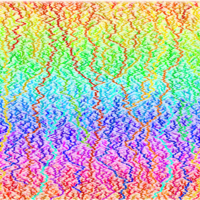# Core Courses#### Students begin their first year with introductory courses in the first 2 weeks (~Sept 20-Oct 1):

Function Spaces and Distribution Theory (Prof. Gui-Qiang G. Chen, 8 hours)

Foundations of Stochastic Analysis (Prof. Ben Hambly, 8 hours)

Foundations of Data Science (Prof. Mihai Cucuringu, 16 hours)

## Advanced Topics in Stochastic Analysis and Control

Wolfgang Stockinger  (Imperial College London)

This course will focus on three main areas:

1. McKean–Vlasov forward-backward stochastic differential equations (SDEs), interacting particle systems, weak convergence of probability measures and Wasserstein metrics

2. Theory and application of mean-field control problems

3. Theory of stochastic integration for processes with jumps, theory of SDEs with jumps.

## Advanced topics in Stochastic Processes

Oana Lang (Imperial College London)

This course will focus on Markov processes and related topics.

We will first review some basic concepts: Markov processes and transition functions; strongly continuous semigroups, generators, and martingale problems; the strong Markov property.

We will then discuss topics chosen from the following list:

• Convergence of stochastic processes
• Well-posedness properties and stochastic flows
• Feynman-Kac formula
• Special classes of processes
• Invariant measures
• Random dynamical systems
• Ergodic theorems for Markov processes

## Theories of Deep LearningProf Jared Tanner (Oxford)

Deep learning is the dominant method for machines to perform classification tasks at reliability rates exceeding that of humans, as well as outperforming world champions in games such as go.

Alongside the proliferating application of these techniques, the practitioners have developed a good understanding of the properties that make these deep nets effective, such as initial layers learning weights similar to those in dictionary learning, while deeper layers instantiate invariance to transforms such as dilation, rotation, and modest diffeomorphisms.

There are now a number of theories being developed to give a mathematical theory to accompany these observations; this course will explore these varying perspectives.

## Simulation Methods and Stochastic AlgorithmsProf. Christoph Reisinger (Oxford)

This course will be an introduction to simulation methods for a wide range of stochastic models. The emphasis is on the construction of the numerical approximations with a limited discussion of their accuracy and computational cost, and almost no stochastic numerical analysis.

The topics covered include:

• random and quasi-random number generation;
• basics of Monte Carlo and Quasi-Monte Carlo simulation;
• Multilevel Monte Carlo and Multilevel QMC methods;
• sensitivity analysis;
• stochastic dierential equations;
• continuous-time Markov processes;
• stochastic PDEs;
• stochastic approximation;
• estimation of invariant measures;
• nested estimation;